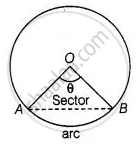# Find the area of the sector of a circle of radius 5 cm, if the corresponding arc length is 3.5 cm. - Mathematics

Sum

Find the area of the sector of a circle of radius 5 cm, if the corresponding arc length is 3.5 cm.

#### Solution

Let the central angle of the sector be θ.

Given that, radius of the sector of a circle (r) = 5 cmAnd arc length (l) = 3.5 cm

∴ Central angle of the sector, theta = ("arc length" (l))/"radius"

⇒ theta = 3.5/5 = 0.7R   .....[because theta = l/r]

⇒ theta = (0.7 xx 180/pi)  .....[because 1R = 180^circ/pi  D^circ]

Now, area of sector with angle θ = 0.7

= (pir^2)/360^circ xx (0.7) xx 180^circ/pi

= (5)^2/2 xx 0.7

= (25 xx 7)/(2 xx 10)

= 175/20

= 8.75 cm2

Hence, the required area of the sector of a circle is 8.75 cm2.

Concept: Areas of Sector and Segment of a Circle
Is there an error in this question or solution?

#### APPEARS IN

NCERT Mathematics Exemplar Class 10
Chapter 11 Area Related To Circles
Exercise 11.4 | Q 8 | Page 133
Share